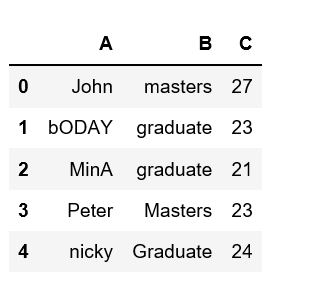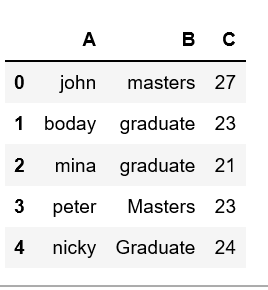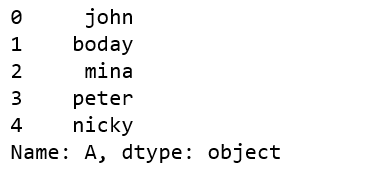# How to lowercase column names in Pandas dataframe

## How to lowercase column names in Pandas dataframe

Analyzing real-world data is somewhat difficult because we need to take various things into consideration. Apart from getting the useful data from large datasets, keeping data in required format is also very important.

One might encounter a situation where we need to lowercase each letter in any specific column in given dataframe. Let’s see how to lowercase column names in Pandas dataframe.

Let’s create a dataframe from the dict of lists.

 `# Create a simple dataframe` `  ` `# importing pandas as pd` `import` `pandas as pd` ` ` `# creating a dataframe` `df ``=` `pd.DataFrame({``'A'``: [``'John'``, ``'bODAY'``, ``'MinA'``, ``'Peter'``, ``'nicky'``],` `                  ``'B'``: [``'masters'``, ``'graduate'``, ``'graduate'``,` `                                   ``'Masters'``, ``'Graduate'``],` `                  ``'C'``: [``27``, ``23``, ``21``, ``23``, ``24``]})` `  ` `df`

Output:There are certain methods we can change/modify the case of column in pandas dataframe. Let’s see how can we lowercase column names in Pandas dataframe using `lower()` method.

Method #1:

 `# Create a simple dataframe` `  ` `# importing pandas as pd` `import` `pandas as pd` ` ` `  ` `# creating a dataframe` `df ``=` `pd.DataFrame({``'A'``: [``'John'``, ``'bODAY'``, ``'MinA'``, ``'Peter'``, ``'nicky'``],` `                  ``'B'``: [``'masters'``, ``'graduate'``, ``'graduate'``, ` `                                   ``'Masters'``, ``'Graduate'``],` `                  ``'C'``: [``27``, ``23``, ``21``, ``23``, ``24``]})` `  ` `df[``'A'``] ``=` `df[``'A'``].``str``.lower()` ` ` `df`

Output:Method #2:

 `# Create a simple dataframe` `  ` `# importing pandas as pd` `import` `pandas as pd` ` ` `  ` `# creating a dataframe` `df ``=` `pd.DataFrame({``'A'``: [``'John'``, ``'bODAY'``, ``'MinA'``, ``'Peter'``, ``'nicky'``],` `                  ``'B'``: [``'masters'``, ``'graduate'``, ``'graduate'``,` `                                   ``'Masters'``, ``'Graduate'``],` `                  ``'C'``: [``27``, ``23``, ``21``, ``23``, ``24``]})` `  ` `df[``'A'``].``apply``(``lambda` `x: x.lower())`

Output:Last Updated on October 23, 2021 by admin

## Pandas Series.rename()Pandas Series.rename()

Python | Pandas Series.rename() Pandas series is a One-dimensional ndarray with axis labels. The labels

## Pandas.date_range() methodPandas.date_range() method

Python | pandas.date_range() method Python is a great language for doing data analysis, primarily because

## Get Cell Value From Pandas DataFrameGet Cell Value From Pandas DataFrame

Pandas is a popular data analysis library in Python. It provides many powerful functions to

## Rename column by index in PandasRename column by index in Pandas

Rename column by index in Pandas A column of a data frame can be changed

## How to Count Distinct Values of a Pandas Dataframe Column?How to Count Distinct Values of a Pandas Dataframe Column?

How to Count Distinct Values of a Pandas Dataframe Column?   Let’s see How to

## How to Convert String to Integer in Pandas DataFrame?How to Convert String to Integer in Pandas DataFrame?

How to Convert String to Integer in Pandas DataFrame? Let’s see methods to convert string